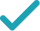Type a math problemSolve for xSteps for Solving Linear Equation
Multiply both sides of the equation by , the least common multiple of .
Use the distributive property to multiply by .
Use the distributive property to multiply by .
Subtract from to get .
Combine and to get .
Use the distributive property to multiply by .
Combine and to get .
Divide both sides by .
GraphGraph Both Sides in 2D
Graph in 2DGiving is as easy as 1, 2, 3
Get 1,000 points to donate to a school of your choice when you join Give With Bing
4\left(5x-1\right)-10\left(1+x\right)=60-5\left(x-1\right)
Multiply both sides of the equation by 20, the least common multiple of 5,2,4.
20x-4-10\left(1+x\right)=60-5\left(x-1\right)
Use the distributive property to multiply 4 by 5x-1.
20x-4-10-10x=60-5\left(x-1\right)
Use the distributive property to multiply -10 by 1+x.
20x-14-10x=60-5\left(x-1\right)
Subtract 10 from -4 to get -14.
10x-14=60-5\left(x-1\right)
Combine 20x and -10x to get 10x.
10x-14=60-5x+5
Use the distributive property to multiply -5 by x-1.
10x-14=65-5x
Add 60 and 5 to get 65.
10x-14+5x=65